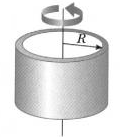Thin Wall Shaft Mass Moment of Inertia

Shaft Cylinder Mass Moment of Inertia Equations and Calculators

The mass moment of inertia, usually denoted I, measures the extent to which an object resists rotational acceleration about an axis, and is the rotational analogue to mass. Mass moments of inertia have units of dimension mass × length2. It should not be confused with the second moment of area, which is used in bending calculations.

Geometrically simple objects have moments of inertia that can be expressed mathematically, but it may not be straightforward to symbolically express the moment of inertia of more complex bodies.

 I = m R2 Where: m = Mass of Shaft Cylinder (lbm , g) R = Radius (in, mm) This expression assumes the shell thickness is negligible. It is a special case of the thick-walled cylindrical tube for R1 = R2. Also, a point mass (m) at the end of a rod of length r has this same moment of inertia and the value R is called the radius of gyration.Thin Wall Cylinder Mass Moment of Inertia Design Variables R (in, mm) = m (lbs, g) = Results I (lbm-in2, g-mm2)Membership Register | LoginHomeEngineering Book StoreEngineering ForumExcel App. DownloadsOnline Books & ManualsEngineering NewsEngineering VideosEngineering CalculatorsEngineering ToolboxGD&T Training Geometric Dimensioning TolerancingDFM DFA TrainingTraining Online EngineeringAdvertising CenterCopyright Notice